# Solving Problems Involving Division of Fractions.pptx

28 de May de 2023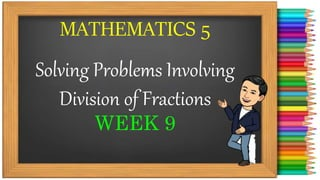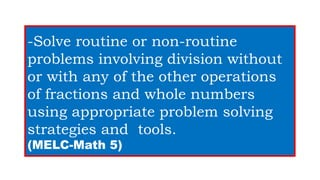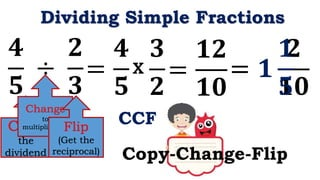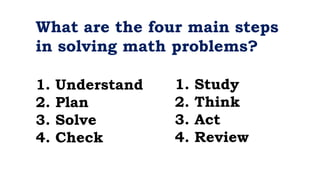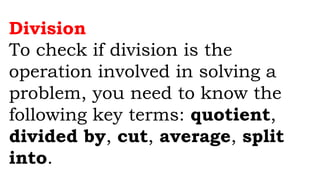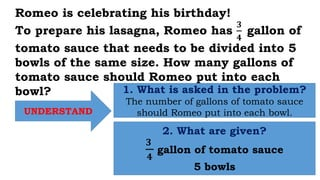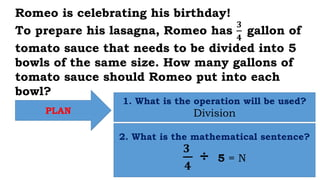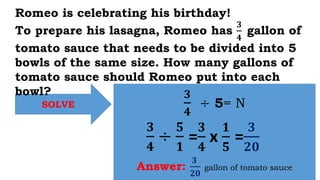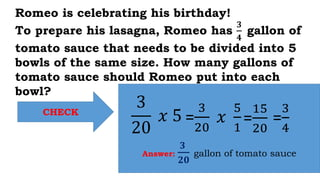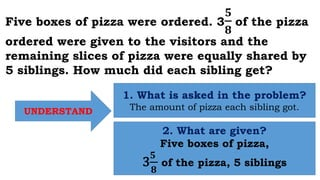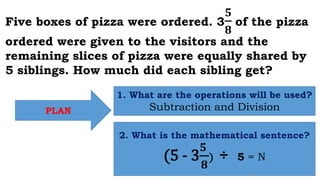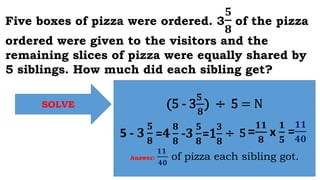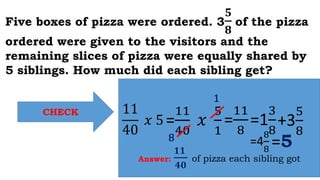1 de 14

### Solving Problems Involving Division of Fractions.pptx

• 1. MATHEMATICS 5 Solving Problems Involving Division of Fractions WEEK 9
• 2. -Solve routine or non-routine problems involving division without or with any of the other operations of fractions and whole numbers using appropriate problem solving strategies and tools. (MELC-Math 5)
• 3. Review
• 4. Dividing Simple Fractions 𝟒 𝟓 ÷ 𝟐 𝟑 = 𝟒 𝟓 CCF Method Copy the dividend Change to multiplication x Flip (Get the reciprocal) 𝟑 𝟐 = 𝟏𝟐 𝟏𝟎 Copy-Change-Flip = 𝟏 𝟐 𝟏𝟎 = 𝟏 𝟏 𝟓
• 5. What are the four main steps in solving math problems? 1. Understand 2. Plan 3. Solve 4. Check 1. Study 2. Think 3. Act 4. Review
• 6. Division To check if division is the operation involved in solving a problem, you need to know the following key terms: quotient, divided by, cut, average, split into.
• 7. Romeo is celebrating his birthday! To prepare his lasagna, Romeo has 𝟑 𝟒 gallon of tomato sauce that needs to be divided into 5 bowls of the same size. How many gallons of tomato sauce should Romeo put into each bowl? UNDERSTAND 1. What is asked in the problem? The number of gallons of tomato sauce should Romeo put into each bowl. 2. What are given? 𝟑 𝟒 gallon of tomato sauce 5 bowls
• 8. PLAN 1. What is the operation will be used? Division 2. What is the mathematical sentence? 𝟑 𝟒 ÷ 5 = N Romeo is celebrating his birthday! To prepare his lasagna, Romeo has 𝟑 𝟒 gallon of tomato sauce that needs to be divided into 5 bowls of the same size. How many gallons of tomato sauce should Romeo put into each bowl?
• 9. SOLVE 𝟑 𝟒 ÷ 5= N Answer: 𝟑 𝟐𝟎 gallon of tomato sauce Romeo is celebrating his birthday! To prepare his lasagna, Romeo has 𝟑 𝟒 gallon of tomato sauce that needs to be divided into 5 bowls of the same size. How many gallons of tomato sauce should Romeo put into each bowl? 𝟑 𝟒 ÷ 𝟓 𝟏 = 𝟑 𝟒 x 𝟏 𝟓 = 𝟑 𝟐𝟎
• 10. CHECK Answer: 𝟑 𝟐𝟎 gallon of tomato sauce Romeo is celebrating his birthday! To prepare his lasagna, Romeo has 𝟑 𝟒 gallon of tomato sauce that needs to be divided into 5 bowls of the same size. How many gallons of tomato sauce should Romeo put into each bowl? 3 20 𝑥 5 = 3 20 𝑥 5 1 = 15 20 = 3 4
• 11. Five boxes of pizza were ordered. 3 𝟓 𝟖 of the pizza ordered were given to the visitors and the remaining slices of pizza were equally shared by 5 siblings. How much did each sibling get? UNDERSTAND 1. What is asked in the problem? The amount of pizza each sibling got. 2. What are given? Five boxes of pizza, 3 𝟓 𝟖 of the pizza, 5 siblings
• 12. PLAN 1. What are the operations will be used? Subtraction and Division 2. What is the mathematical sentence? (5 - 3 𝟓 𝟖 ) ÷ 5 = N Five boxes of pizza were ordered. 3 𝟓 𝟖 of the pizza ordered were given to the visitors and the remaining slices of pizza were equally shared by 5 siblings. How much did each sibling get?
• 13. SOLVE (5 - 3 𝟓 𝟖 ) ÷ 5 = N Answer: 𝟏𝟏 𝟒𝟎 of pizza each sibling got. 5 - 𝟑 𝟓 𝟖 =𝟒 𝟖 𝟖 -𝟑 𝟓 𝟖 = 𝟏𝟏 𝟖 x 𝟏 𝟓 = 𝟏𝟏 𝟒𝟎 Five boxes of pizza were ordered. 3 𝟓 𝟖 of the pizza ordered were given to the visitors and the remaining slices of pizza were equally shared by 5 siblings. How much did each sibling get? =1 𝟑 𝟖 ÷ 5
• 14. CHECK Answer: 𝟏𝟏 𝟒𝟎 of pizza each sibling got 11 40 𝑥 5= 11 40 𝑥 5 1 = 11 8 =1 3 8 Five boxes of pizza were ordered. 3 𝟓 𝟖 of the pizza ordered were given to the visitors and the remaining slices of pizza were equally shared by 5 siblings. How much did each sibling get? 1 8 +3 5 8 =4 8 8 =5